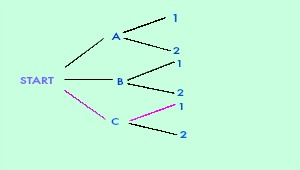# Tree Diagrams in Math: Definition & Examples - Quiz & Worksheet

Instructions:

question 1 of 3

### How many outfits could you make if you had three pairs of pants, three shirts, and two pairs of shoes?

Create Your Account To Take This Quiz

As a member, you'll also get unlimited access to over 84,000 lessons in math, English, science, history, and more. Plus, get practice tests, quizzes, and personalized coaching to help you succeed.

Try it risk-free for 30 days. Cancel anytime

### 2. The following tree diagram represents the possible outcomes of a certain event. What outcome is represented by the path marked with pink branches?Create your account to access this entire worksheet
Quizzes, practice exams & worksheets
Certificate of Completion
Create an account to get started

You can build a better conceptual understanding of tree diagrams in math with this worksheet and quiz. Topics you'll need to address include how many outfits you'd be able to make with a given amount of articles of clothing and the number of possible outcomes with a six-sided die.

## Quiz & Worksheet Goals

The goals of the quiz and worksheet are to get you to:

• Determine the outcome represented by a given example of a tree diagram
• Name the number of possible outcomes for a certain event represented by a tree diagram
• Apply the theories of the tree diagram in real life situations

## Skills Practiced

With the quiz and worksheet you will practice the following:

• Making connections - use understanding of the concept of possible outcomes and how they are connected to tree diagrams
• Interpreting information - verify that you can read information regarding a six-sided die and interpret it correctly
• Information recall - access the knowledge you've gained regarding tree diagrams

Utilize this lesson entitled Tree Diagrams in Math: Definition & Examples for more information on the subject. Once you've completed the lesson, you will have learned the following study objectives:

• Understand the purpose of a tree diagram
• Analyze examples
• Recognize the different situations a tree diagram can be used for
Final ExamCollege Preparatory Mathematics: Help and Review
Status: Not Started
Chapter ExamBasic Probability and Statistics: Help and Review
Status: Not Started

Support